# MAXIFS & MINIFS Functions – Get Max / Min If Condition is met – Excel & Google Sheets

This tutorial demonstrates how to use the Excel MAXIFS  and MINIFS Functions in Excel to count data that meet certain criteria.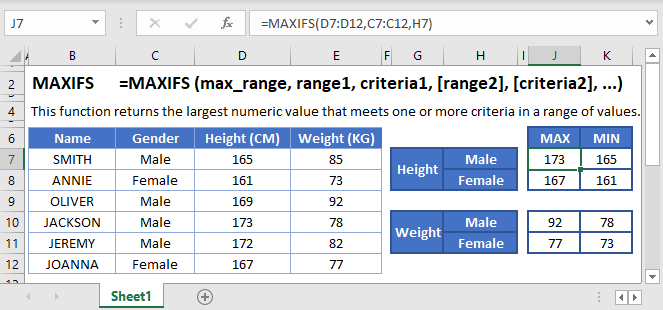## MAXIFS Function Overview

You can use the MAXIFS function in Excel to count cells that contain a specific value, count cells that are greater than or equal to a value, etc.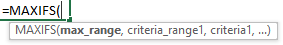(Notice how the formula inputs appear)

## MAXIFS Function Syntax and Arguments:

``=MAXIFS(max_range, range1, criteria1, [range2], [criteria2], ...)``

max_range – Range of values used to determine maximum.

range1 – The first range to evaluate.

criteria1 – The criteria to use on range1.

range2 – [optional] The second range to evaluate.

range2 – [optional] The second range to evaluate.

## What are the MAXIFS and MINIFS functions?

While Microsoft was able to give us early on a way to find the sum or count of a range of cells based on criteria, the MAXIFS and MINIFS came later in the life of spreadsheets. Thankfully, we have them now and they have very similar structure and use. Simply put, the two functions can scan through a range of cells checking for a specific criterion, and then giving the max or of the values in a range that correspond to those values. Because they were created after the big 2007 update, there is no older “MAXIF/MINIF” to worry about.

If you haven’t already, you can review much of the similar structure and examples in the COUNTIFS article <insert link>.

### Basic example

Let’s consider this table: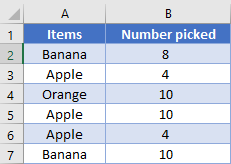If we want to find what was the maximum number of bananas picked, we can use the MAXIFS function. We’ll always give the range of numbers we want to return the result from as the first argument. Then, we’ll list the criteria ranges and their corresponding criteria. In our example, we can place this formula in D2:

``=MAXIFS(B2:B7, A2:A7, D1)``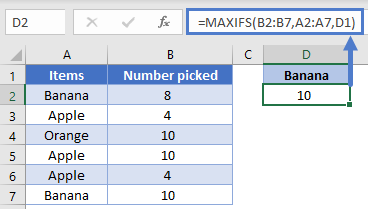Similarly, if we wanted to find the smallest/minimum number of bananas picked, we can simply switch the name of the function while keeping the rest the same.

``=MINIFS(B2:B7, A2:A7, D1)``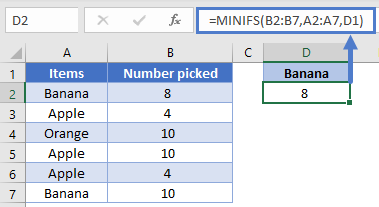For the rest of the article, we’ll tend to present examples using either MAXIFS or MINIFS rather than listing both each time. Just remember that you can easily switch between the two depending on which result you’re trying to achieve.

### Working with Dates, Multiple criteria

When working with dates in a spreadsheet, while it is possible to input the date directly into the formula, it’s best practice to have the date in a cell so that you can just reference the cell in a formula. For example, this helps the computer know that you’re wanting to use the date 5/27/2020, and not the number 5 divided by 27 divided by 2020.

Let’s look at our next table recording the number of visitors to a site every two weeks.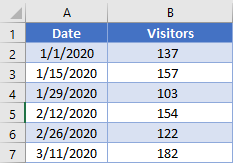We can specify the start and end points of the range we want to look at in D2 and E2. Our formula then to find the highest number of visitors in this range could be:

``=MAXIFS(B2:B7, A2:A7, ">="&D2, A2:A7, "<="&E2)``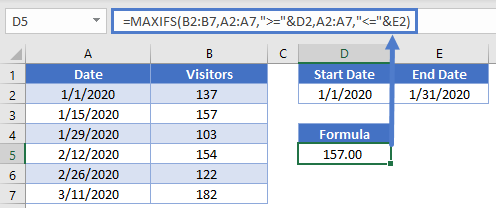Note how we were able to concatenate the comparisons of “<=” and “>=” to the cell references to create the criteria. Also, even though both criteria were being applied to the same range of cells (A2:A7), you need to write out the range twice, once per each criterion.

### Multiple columns

When using multiple criteria, you can apply them to the same range as we did with previous example, or you can apply them to different ranges. Let’s combine our sample data into this table: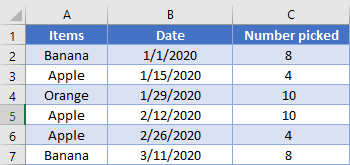We’ve setup some cells for the user to enter what they want to search for in cells E2 through G2. We thus need a formula that will add up the fewest number of apples picked in February. Our formula looks like this:

=MINIFS(C2:C7, B2:B7, “>=”&F2, B2:B7, “<=”&G2, A2:A7, E2)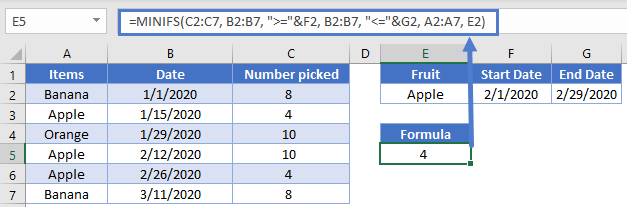### Easy Excel Automation### MAXIFS/MINIFS with OR type logic

Up to this point, the examples we’ve used have all been AND based comparison, where we are looking for rows that meet all our criteria. Now, we’ll consider the case when you want to search for the possibility of a row meeting one or another criterion.

Let’s look at this list of sales: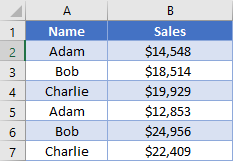We’d like to find the max sales for both Adam and Bob. The simplest is to take two MAXIFS, and then take the MAX of both of those functions.

``=MAX(MAXIFS(B2:B7, A2:A7, "Adam"),  MAXIFS(B2:B7, A2:A7, "Bob"))``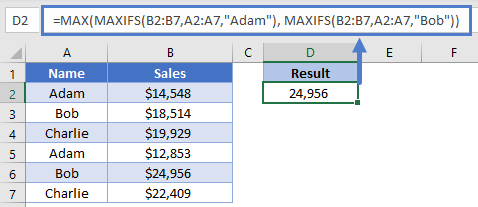Here, we’ve had the computer calculate our individual scores, and then we add them together.

Our next option is good for when you have more criteria ranges, such that you don’t want to have to rewrite the whole formula repeatedly. In the previous formula, we manually told the computer to calculate two different MAXIFS. However, you can also do this by writing your criteria inside an array, like this:

``=MAX(MAXIFS(B2:B7, A2:A7, {"Adam", "Bob"}))``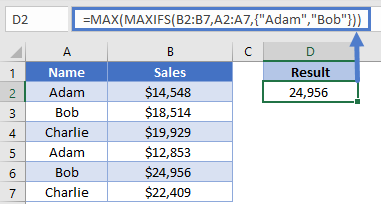Look at how the array is constructed inside the curly brackets. When the computer evaluates this formula, it will know that we want to calculate a MAXIFS function for each item in our array, thus creating an array of numbers. The outer MAX function will then take that array of numbers and turn it into a single number. Stepping through the formula evaluation, it would look like this:

``````=MAX(MAXIFS(B2:B7, A2:A7, {"Adam", "Bob"}))
=MAX(14548, 24956)
=24956``````

We get the same result, but we were able to write out the formula a bit more succinctly.

### Dealing with blanks

Sometimes your data set will have blank cells that you need to either find or avoid. Setting up the criteria for these can be a little tricky, so let’s look at another example.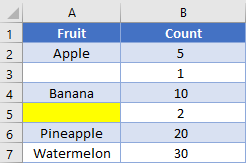Note that cell A3 is truly blank, while cell A5 has a formula returning a zero-length string of “”. If we want to find the total average of truly blank cells, we’d use a criterion of “=”, and our formula would look like this:

``=MAXIFS(B2:B7,A2:A7,"=")``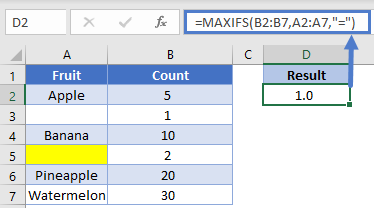On the other hand, if we want to get the average for all cells that visually looks blank, we’ll change the criteria to be “”, and the formula looks like

``=MAXIFS(B2:B7,A2:A7,"")``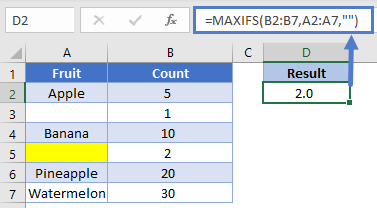Let’s flip it around: what if you want to find the average of non-blank cells? Unfortunately, the current design won’t let you avoid the zero-length string. You can use a criterion of “<>”, but as you can see in the example, it still includes the value from row 5.

``````=MAXIFS(B2:B7,A2:A7,"<>")
``````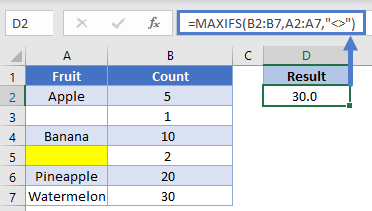If you need to not count cells containing zero length strings, you’ll want to consider using the LEN function inside a SUMPRODUCT <link to SUMPRODUCT article).

## MAXIFS & MINIIFS in Google Sheets

The MAXIFS & MINIIFS Function works exactly the same in Google Sheets as in Excel: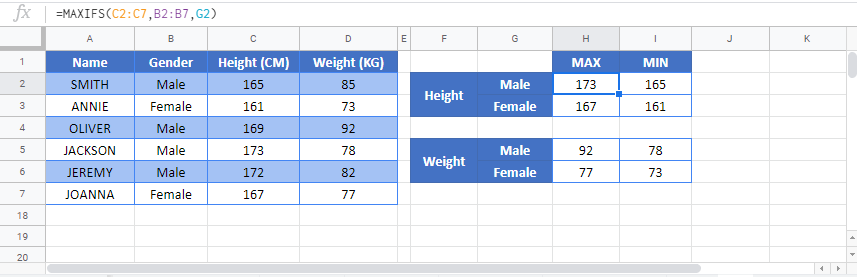### Excel Practice Worksheet

Practice Excel functions and formulas with our 100% free practice worksheets!